# Hölder summation methods

(diff) ← Older revision | Latest revision (diff) | Newer revision → (diff)

A collection of methods for summing series of numbers, introduced by O. Hölder  as a generalization of the summation method of arithmetical averages (cf. Arithmetical averages, summation method of). The series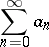is summable by the Hölder methodto sumif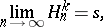where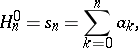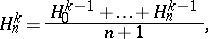. In particular,-summability of a series indicates that it converges in the ordinary sense;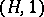is the method of arithmetical averages. The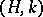-methods are totally regular summation methods for anyand are compatible for all(cf. Compatibility of summation methods). The power of the method increases with increasing: If a series is summable to a sumby the method, it will also be summable to that sum by the method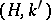for any. For anythe methodis equipotent and compatible with the Cesàro summation method of the same order(cf. Cesàro summation methods). If a series is summable by the method, its termsnecessarily satisfy the condition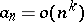.

How to Cite This Entry:
Hölder summation methods. Encyclopedia of Mathematics. URL: http://encyclopediaofmath.org/index.php?title=H%C3%B6lder_summation_methods&oldid=18982
This article was adapted from an original article by I.I. Volkov (originator), which appeared in Encyclopedia of Mathematics - ISBN 1402006098. See original article